# API Reference¶

The namespace of scikit-guess is called skg. It exports all of the parameter estimation functions provided by the scikit.

The functions themselves live in individual sub-modules. Each module containing a fitting function also defines a model attribute, which refers to the model function being fitted. Each fitting routine has a model attribute pointing to the module-level model.

Sub-modules

 exp Exponential fit with and additive bias of the form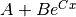. gauss_cdf Gaussian cumulative distribution fit. gauss_pdf Gaussian probability density fit. gauss Unnormalized Gaussian bell curve fit. pow Power fit with additive bias of the form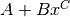. weibull_cdf Weibull cumulative distribution fit with three parameters, of the form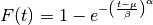. ngauss N-dimensional, unnormalized Gaussian fit. nsphere N-sphere fit with center and radius. util Shared utility functions used by the fitting routines.

Exported Functions

These functions are available directly in the skg namespace in addition to the modules that define them.

 exp_fit(x, y[, sorted]) Exponential fit of the form. gauss_cdf_fit(x, y[, sorted]) Gaussian CDF fit of the form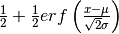. gauss_pdf_fit(x, y[, sorted]) Gaussian PDF fit of the form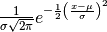. gauss_fit(x, y[, sorted]) Gaussian bell curve fit of the form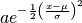. pow_fit(x, y[, sorted]) Power fit of the form. weibull_cdf_fit(x, y[, sorted]) Weibull CDF fit of the form. ngauss_fit(x, y[, axis, weights, scaling]) An N-dimensional Gaussian fit. ngauss_from_image(img[, weights, scaling]) Compute a Gaussian fit to an entire image. nsphere_fit(x[, axis, scaling]) Fit an n-sphere to ND data.